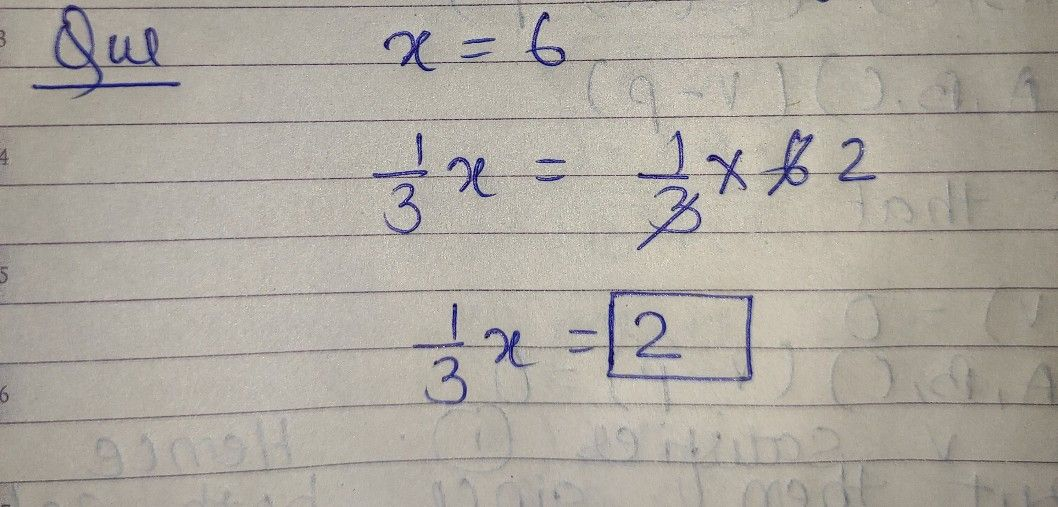Symbol
ProblemQuestion $4$ If $x$ is $6$ what $|s$ $\dfrac {1} {3}x$ $1frac\left(1\right)\left(3\right)x$